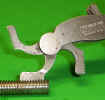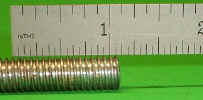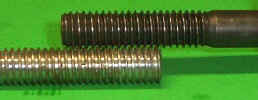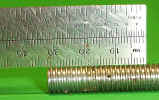Home

Measuring and Defining Thread Size

Fasteners (screws, nuts & bolts) hold most of what we know in guns and life together.  The following is what I explain to my students how screw threads are described.

Screw threads have two descriptions.  One is its diameter, measured to the height of the threads.  The other is how many threads per inch.  Or, in metric use, the pitch of the threads that is the measurement from one crest to the next.

The diameter, the outside size of the fastener, measured fractionally for the inch system and by millimeters for the metric system has an exception in the inch system.  Under a quarter inch diameter the inch system uses fractional and number sizes.  Here’s a list of the number sizes with the nominal standard size of the screw:

#3 would be .097 diameter

#4                .110

#5                .122

#6                .135

#8                .160

#10              .187

#12              .215

#14              .240

A number size screw, written by diameter and thread pitch, would be 6-48 (scope base screw size); 6 being the decimal equivalent and 48 being the number of threads per inch.
Screws are not the full dimension of their description.  An example is
a 3/8-diameter thread will not measure exactly .375, but will be some thousandths smaller.  This is because the sharp “V” of the thread has been eliminated.  There’s no strength in the thin crest of the “V” and this very thin section could be damaged in handling.

To determine the number of threads per inch, a machine shop would use a thread pitch gauge.  The gauge has multiple blades, each with a different thread pitch on it, and using it like a comb you use the blade that fits the thread. When you find the number of threads per inch, a fractional screw size would be written: diameter-threads per inch. Example: 1/2-20, 1/2 (inch) being the diameter and 20 threads per inch.click on pictures to enlarge

Most hobbyists don’t have a thread pitch gauge, so you can use a scale.  You can count how many thread crests are in an inch.  If the fastener is short, measure how many in a quarter inch, then multiply by 4.Another method of determining the thread pitch is to use a known thread on a screw or bolt, like you would use a gauge.  An example is:  if you had a 10-32 screw, you can use it to check any other 32-pitch thread, such as ¼-32 or ½-32, or any other 32 thread per inch screw.Metric fasteners can be measured as above, but the metric description uses the distance between thread crest to thread crest.  When using a scale the thread may be finer than the graduations on your scale.  So count how many threads are in a centimeter and divide by 10.  Or, if the screw is shorter than a centimeter, count the amount in half a centimeter and divide by 5.  The screw size would be written diameter in millimeters x distance from crest to crest in millimeter. An example would be 8 x 1; so the bolt would be 8mm dia. and 1mm from thread crest to crest.click on pictures to enlarge